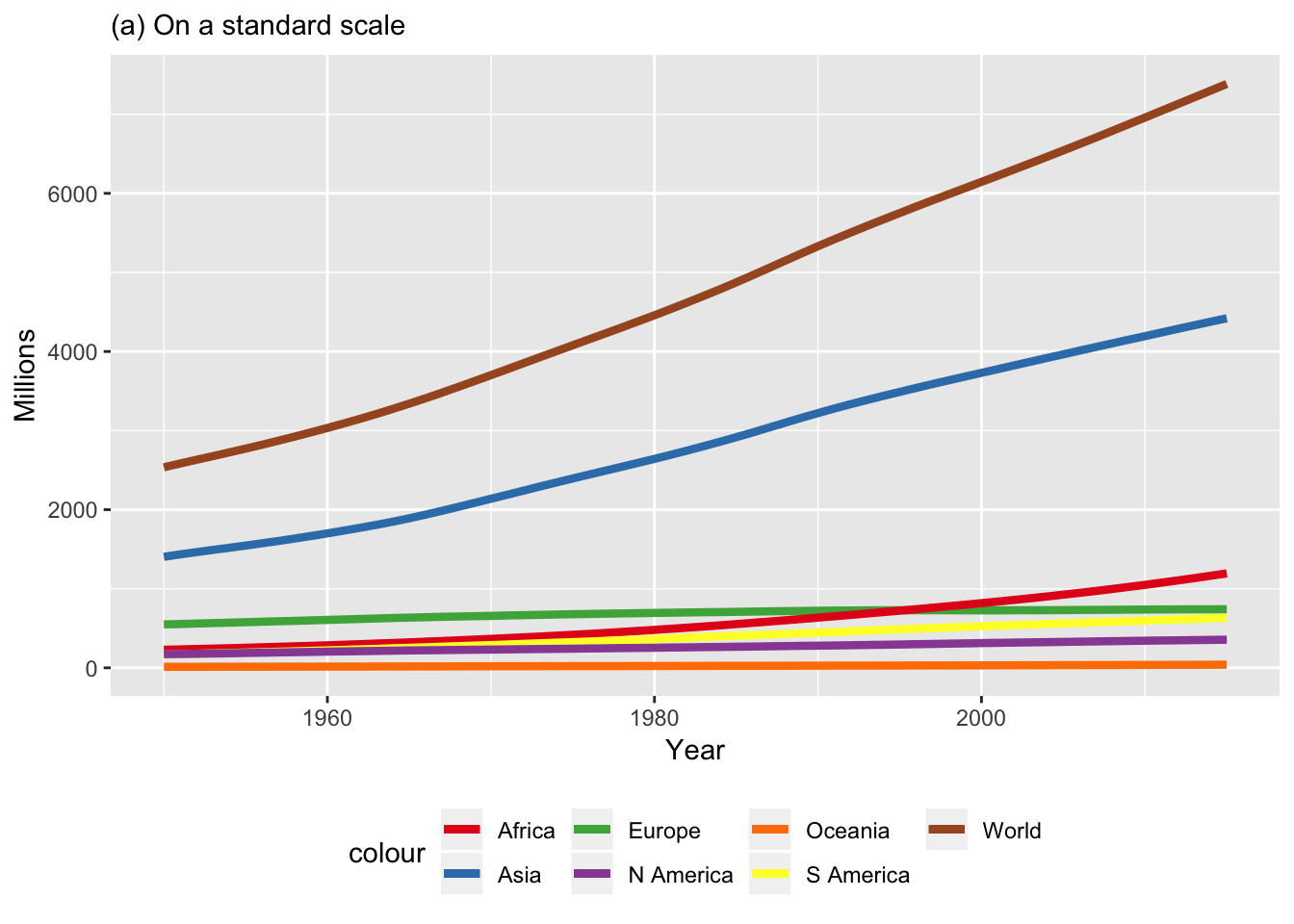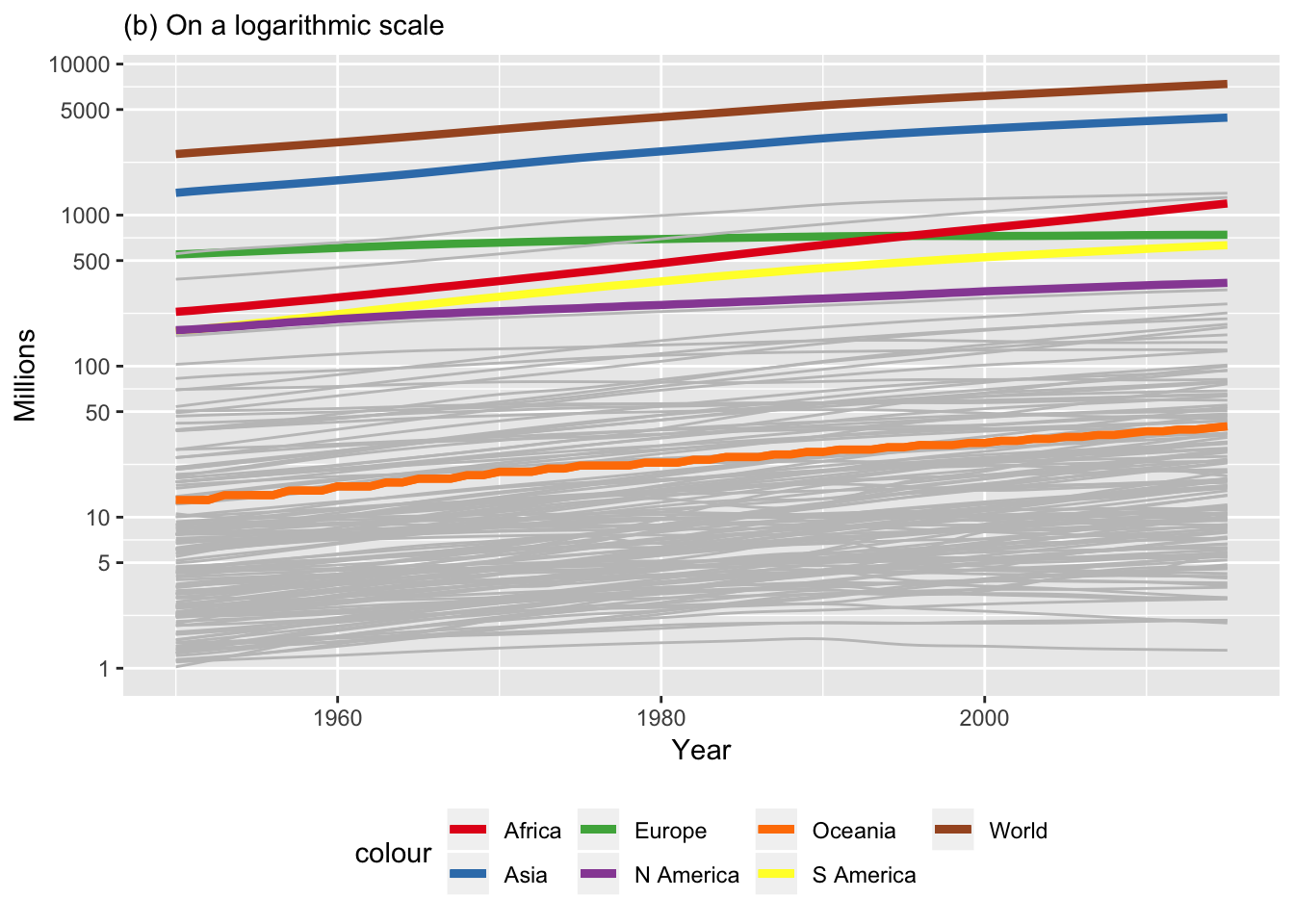Data is from United Nations Population Division: World Population Prospects 2017, specifically Total Population - Both Sexes

library(ggplot2)

p <- ggplot(popTrends, aes(x=Year, y)) # constructs initial plot object, p
p <- p + geom_line(aes(y = Europe, col = "Europe"), size=1.5) # adds a y-series
p <- p + geom_line(aes(y = World, col = "World"), size=1.5) # adds a y-series
p <- p + geom_line(aes(y = Africa, col = "Africa"), size=1.5) # adds a y-series
p <- p + geom_line(aes(y = Oceania, col = "Oceania"), size=1.5) # adds a y-series
p <- p + geom_line(aes(y = Asia, col = "Asia"), size=1.5) # adds a y-series
p <- p + geom_line(aes(y = LatinAmericaandCarribean, col = "S America"), size=1.5) # adds a y-series
p <- p + geom_line(aes(y = NorthernAmerica, col = "N America"), size=1.5) # adds a y-series
p <- p +  labs(subtitle="(a) On a standard scale", y="Millions", x="Year") # Adds title, subtitle

# Some of the colours rather pale to see in palette Accent, Set1 seems better
p <- p + scale_colour_brewer(palette = "Set1")
#p <- p + scale_colour_brewer(palette = "Accent")

p <- p  + scale_size_continuous(name = "Size", guide = FALSE)  # turns off unwanted size legend
p <- p + theme(legend.position="bottom", legend.box = "horizontal") # positions the colour legend
pFigure 2.7(a) . Total population for the world and continents between 1950 and 2015, both sexes combined.

# now for the logarithmic version

logbreaks <- c(1, 5, 10, 50, 100, 500, 1000, 5000, 10000)
q <- p +  labs(subtitle="(b) On a logarithmic scale", y="Millions", x="Year") # reword title, subtitle, and caption

# try trick from https://www.biostars.org/p/234142/  using aes_string?

plotIt <- function(q, index){ #recursive function to draw lines for countries that had at least a million population in 1951
if(index==1){return(q)}else{ #end recursion when index reaches 1
q <- q + geom_line(aes(y=millIn51[,index]/1000), col = "grey76", size=0.5) # or add the y-series
plotIt(q, index-1) # and resursively call next index
}
}
q <- plotIt(q, 141) # call recursive function for the 140 countries in the CSV (recursion ends when index reaches 1, the x=Year field)
q <- q + geom_line(aes(y = Oceania, col = "Oceania"), size=1.5) # for visibility put Oceania back on top of the sea of grey
q <- q + scale_y_log10(breaks=logbreaks) # change to logarithmic y-scale
q <- q + theme(legend.position="bottom", legend.box = "horizontal")
qFigure 2.7(b) . Total population for the world and continents between 1950 and 2015, both sexes combined, on a logarithmic scale, with the trend-lines for individual countries that had a population of at least one million in 1951.